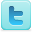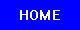Density of Various Materials
K-12 Experiments & Background information
For Science Labs, Lesson Plans, Class Activities & Science Fair Projects
For Middle School Students & Teachers

 This experiment is courtesy of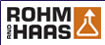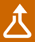## Get Down With Density

Developers:Barbara Allen
Beeber Middle School

Denis Dollard
Analytical Research
Rohm and Haas Company
Bristol, PA

Level:6 and 7

Objectives:

1. Students will be able to define density.

2. Students will observe that things that are less dense than water will float.

3. Students will be able to find the density of copper, iron and aluminum.

Vocabulary:

DENSITY = the mass per unit volume of a substance.

MASS = the amount of matter in an object.

VOLUME = the amount of space something takes up.

ELEMENT = a simple substance that cannot be broken down into simpler substances.

Background:

Density is a basic physical property of all matter. Every substance has a density that can be measured and the density of a substance is always the same. If you were asked, which do you think is heavier, a kilogram of feathers or a kilogram of lead, your initial response would probably be the lead. Surprisingly, they both weigh the same amount. How is this possible? A kilogram of feathers takes up a large amount of space, or volume. A kilogram of lead is small enough to hold in your hand. The kilogram of lead takes up less space because lead has a much greater density than the feathers. Density is the mass per unit volume of a substance. To find the density of a substance divide the mass by the volume.

 Density = Mass _______ Volume

The amount of matter in an object is its mass. Consider the matter in a bag of potatoes to that of a bag of popcorn. The bag of potatoes has more matter than the bag of popcorn. The bag of potatoes has more mass. Mass is measured with an instrument called a balance, in units of grams. The amount of space an object takes up is called its volume. A graduated cylinder is used to measure the volume of a liquid and is often measured in milliliters. The volume of a solid can be determined by measuring the amount of water the solid displaces in a graduated cylinder.

Lesson:

Materials:Density of Metals

iron cylinder (Fe - #26)
aluminum cylinder (Al - #13)
water (H
20)
unknown sample (brass)
copper cylinder (Cu - #29)
triple beam balance

Procedure:

1. Weigh the three samples on a triple beam balance, to find the mass.
3. Fill a 25-ml graduated cylinder with 10 ml of water.
4. Tilt the graduated cylinder slightly and place one sample in the graduated cylinder. Allow the sample to slide down the side of the cylinder. Try to avoid any water splashing or spillage.
5. Record the volume of the water in the cylinder with the metal in it on the worksheet.
6. Subtract the volume of the water from the volume of the metal and water, to get the volume of the metal.
7. Remove water and sample from cylinder.
8. Repeat steps 3-7 for the two remaining samples.
9. Calculate the density of the metals examined on the worksheet.

Questions:

1. Which metal is the most dense?
2. Which metal is the least dense?
3. The density of water is 1 gram per milliliter. If ice floats on water, predict what you would think the density of an ice cube in a glass of water would be.

Explanation:The density of water is 1 gram per milliliter. The metals used in this experiment have a density greater than 1 g/ml, therefore all the metals sink in water.

Follow-Up Activities:

Worksheet #2

References:

Bernstein, L., Schachter, M., Winkler, A., & Wolfe, S. (1991 ) Concepts and Challenges in Physical Science, Globe Book Company. Englewood Cliffs. New Jersey.

Kellerman, Elizabeth R., Simple Chemistry, Grosset and Dunlap Publishers, New York: 1966.

Palder, Edward L., Magic With Chemistry, Milliken Publishing Co., St. Louis, Missouri: 1984.

Weast, R.C., CRC Handbook of Chemistry and Physics, 62nd Edition, CRC Press, Inc., Boca Raton, Florida: 1982

Worksheets: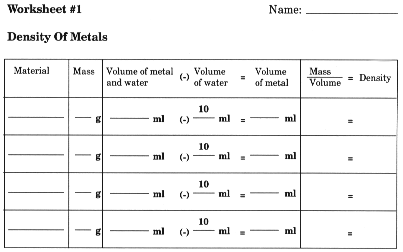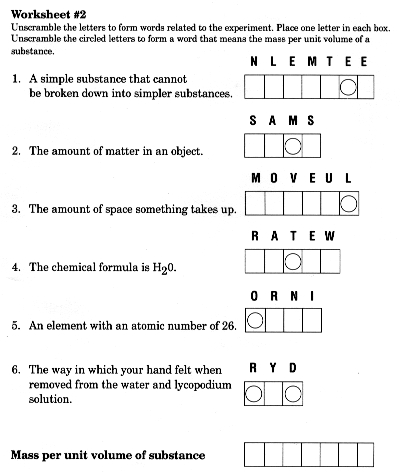This experiment is courtesy of